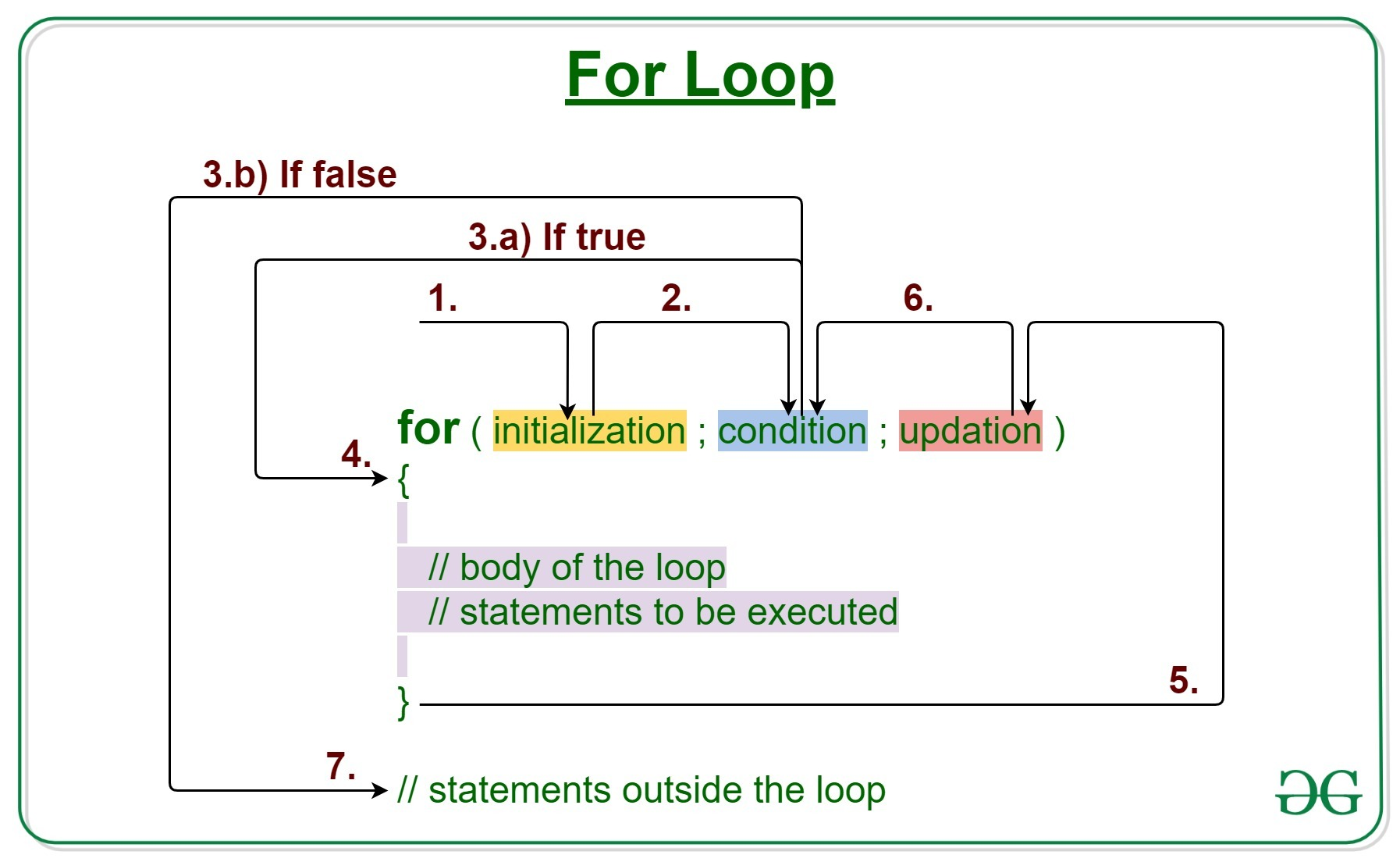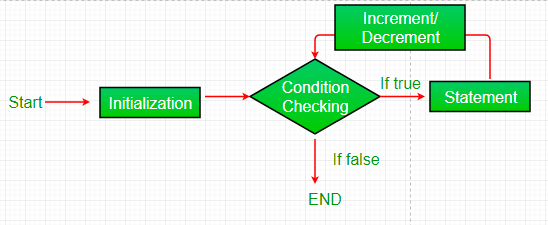Skip to content
Related Articles
Java For loop with Examples
• Difficulty Level : Medium
• Last Updated : 17 Feb, 2021

Loops in Java come into use when we need to repeatedly execute a block of statements.

Java for loop provides a concise way of writing the loop structure. The for statement consumes the initialization, condition and increment/decrement in one line thereby providing a shorter, easy to debug structure of looping.Syntax:

```for (initialization expr; test expr; update exp
{
// body of the loop
// statements we want to execute
}
```

The various parts of the For loop are:

1. Initialization Expression: In this expression we have to initialize the loop counter to some value.
Example:
`int i=1;`
2. Test Expression: In this expression we have to test the condition. If the condition evaluates to true then we will execute the body of the loop and go to update expression. Otherwise, we will exit from the for loop.
Example:
`i <= 10`
3. Update Expression: After executing the loop body, this expression increments/decrements the loop variable by some value.
Example:
`i++;`

How does a For loop executes?

1. Control falls into the for loop. Initialization is done
2. The flow jumps to Condition
3. Condition is tested.
1. If Condition yields true, the flow goes into the Body
2. If Condition yields false, the flow goes outside the loop
4. The statements inside the body of the loop get executed.
5. The flow goes to the Updation
6. Updation takes place and the flow goes to Step 3 again
7. The for loop has ended and the flow has gone outside.

Flow chart for loop (For Control Flow):Example 1: This program will try to print “Hello World” 5 times.

 `// Java program to illustrate for loop``class` `forLoopDemo {``    ``public` `static` `void` `main(String args[])``    ``{``        ``// Writing a for loop``        ``// to print Hello World 5 times``        ``for` `(``int` `i = ``1``; i <= ``5``; i++)``            ``System.out.println(``"Heelo World"``);``    ``}``}`
Output:
```Heelo World!
Heelo World!
Heelo World!
Heelo World!
Heelo World!
```

Dry-Running Example 1: The program will execute in the following manner.

```1. Program starts.
2. i is initialized with value 1.
3. Condition is checked. 1 <= 5 yields true.
3.a) "Hello World" gets printed 1st time.
3.b) Updation is done. Now i = 2.
4. Condition is checked. 2 <= 5 yields true.
4.a) "Hello World" gets printed 2nd time.
4.b) Updation is done. Now i = 3.
5. Condition is checked. 3 <= 5 yields true.
5.a) "Hello World" gets printed 3rd time
5.b) Updation is done. Now i = 4.
6. Condition is checked. 4 <= 5 yields true.
6.a) "Hello World" gets printed 4th time
6.b) Updation is done. Now i = 5.
7. Condition is checked. 5 <= 5 yields true.
7.a) "Hello World" gets printed 5th time
7.b) Updation is done. Now i = 6.
8. Condition is checked. 6 <= 5 yields false.
9. Flow goes outside the loop. Program terminates.
```

Example 2: The following program prints the sum of x ranging from 1 to 20.

 `// Java program to illustrate for loop.``class` `forLoopDemo {``    ``public` `static` `void` `main(String args[])``    ``{``        ``int` `sum = ``0``;`` ` `        ``// for loop begins``        ``// and runs till x <= 20``        ``for` `(``int` `x = ``1``; x <= ``20``; x++) {``            ``sum = sum + x;``        ``}``        ``System.out.println(``"Sum: "` `+ sum);``    ``}``}`
Output:
```Sum: 210
```

Enhanced For Loop or Java For-Each loop

Java also includes another version of for loop introduced in Java 5. Enhanced for loop provides a simpler way to iterate through the elements of a collection or array. It is inflexible and should be used only when there is a need to iterate through the elements in a sequential manner without knowing the index of the currently processed element.

Note: The object/variable is immutable when enhanced for loop is used i.e it ensures that the values in the array can not be modified, so it can be said as a read-only loop where you can’t update the values as opposed to other loops where values can be modified.
Syntax:

```for (T element:Collection obj/array)
{
// loop body
// statement(s)
}```

Let's take an example to demonstrate how enhanced for loop can be used to simplify the work. Suppose there is an array of names and we want to print all the names in that array. Let’s see the difference between these two examples by this simple implementation:

 `// Java program to illustrate enhanced for loop`` ` `public` `class` `enhancedforloop {`` ` `    ``public` `static` `void` `main(String args[])``    ``{``        ``String array[] = { ``"Ron"``, ``"Harry"``, ``"Hermoine"` `};`` ` `        ``// enhanced for loop``        ``for` `(String x : array) {``            ``System.out.println(x);``        ``}`` ` `        ``/* for loop for same function ``        ``for (int i = 0; i < array.length; i++) ``        ``{ ``            ``System.out.println(array[i]); ``        ``} ``        ``*/``    ``}``}`
Output:
```Ron
Harry
Hermoine
```

Recommendation: Use this form of the statement instead of the general form whenever possible. (as per JAVA doc.)

Related Articles:

Attention reader! Don’t stop learning now. Get hold of all the important Java Foundation and Collections concepts with the Fundamentals of Java and Java Collections Course at a student-friendly price and become industry ready. To complete your preparation from learning a language to DS Algo and many more,  please refer Complete Interview Preparation Course.

My Personal Notes arrow_drop_up